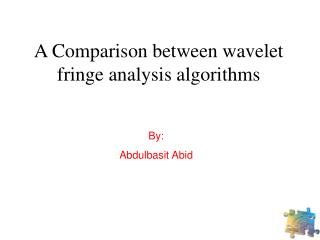DownloadDownload PresentationA Comparison between wavelet fringe analysis algorithms

# A Comparison between wavelet fringe analysis algorithms

Download Presentation## A Comparison between wavelet fringe analysis algorithms

- - - - - - - - - - - - - - - - - - - - - - - - - - - E N D - - - - - - - - - - - - - - - - - - - - - - - - - - -
##### Presentation Transcript

1. A Comparison between wavelet fringe analysis algorithms By: Abdulbasit Abid

2. Outline • Fringe Analysis. • Wavelet Transform (WT). • Wavelet fringe analysis algorithms • Computer simulated & real fringes. • Comparison

3. CCD Camera Fringe Analysis: Fringes Object Fringe pattern • A structured light fringe pattern is projected onto the object’s surface. • The projected fringe pattern is phase modulated by the object heights. • The image of the deformed fringe pattern is captured by a CCD camera and stored in the computer.

4. Wavelet Wavelet: • Means a small wave of limited duration (with zero average and finite energy). • It could be real or complex. Examples of wavelets: Morlet Mexican hat Gaussian

5. Wavelet transform A modulated signal The result is a time-scale plot

6. Wavelet fringe analysis algorithms Frequency estimation Phase estimation Estimates the instantaneous frequencies, which are integrated to estimate the phase Estimates the phase Suffers from 2π discontinuities Phase unwrapping is not required Phase unwrapping is required Employs complex and real wavelet Employs complex wavelet

7. Simulated fringes Fringe Analysis: Phase estimation 2. The result is 1. Apply WT to the first row of the fringes (using complex Morlet wavelet). 2D Complex Array 64 512

8. 3. Find the maximum value in each column of the Modulus array. 4. Find the corresponding angle from the Phase array.

9. 5. If we repeat the previous process for all the 512 rows, we will get a wrapped phase. 6. Therefore, an unwrapping algorithm is needed

10. Simulated fringes Fringe Analysis: Frequency estimation 2. The result is 1. Apply WT to the first row of the fringes (using complex Morlet wavelet). 2D Complex Array 64 512

11. 3. Find the maximum value in each column of the Modulus array. 4. Find the corresponding scale values at these maximum values Scales 5. Find the instantaneous frequency by:

12. 6. Finally, the phase distribution can be found by integrating the estimated frequencies. Therefore phase unwrapping is not required

13. Real fringes Fringe Analysis: Phase estimation

14. Real fringes Fringe Analysis: Frequency estimation

15. Comparison

16. Questions?